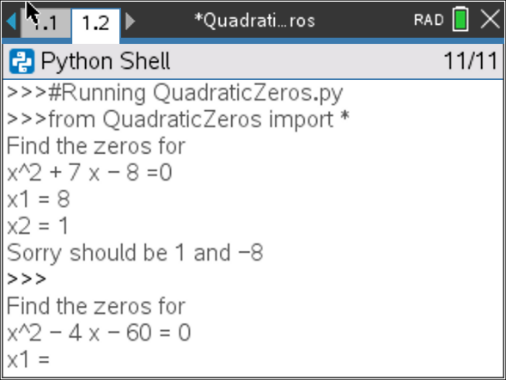# Activities

•6-8
9-12

50 Minutes

• ##### Device
•TI-Nspire™ CX II
•TI-Nspire™ CX II CAS
• ##### Software

TI-Nspire™ CX
TI-Nspire™ CX CAS

5.2

• ##### Report an Issue#### Activity Overview

In this coding activity, students will explore factored equations, and create a game to practice finding integer x-intercepts for equations.

#### Objectives

• Use the input function and a variable to collect and store data from a user
• Use the randint() function to generate random integers.
• Use a while loop to repeat code
• Use if..elif..else statements to make decisions.
• Explore how x-intercepts are related to factored quadratic equations
• Explore how to factor equation in standard form
• Factor quadratic equations with integer solutions

In this lesson, students will learn how to find the x-intercepts for equations in the form y = (x – x 1)(x – x 2).

Students will explore how factored equations in the form
y = (x – x 1)(x – x 2) can be rewritten in a standard form
y = x2 + bx + c.

Students will create a game that will let you practice finding integer x-intercepts for equations of the form
y = x2 + bx + c.

Note: This lesson requires the use of TI-Nspire™ CX II technology with OS 5.2 and above.